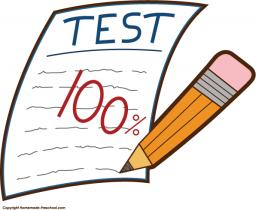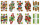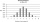# Test

The teacher prepared a test with ten questions. The student has the option to choose one correct answer from the four (A, B, C, D). The student did not get a written exam at all. What is the probability that:

Correct result:

a =  0.0584
b =  0
c =  0.0563

#### Solution:We would be pleased if you find an error in the word problem, spelling mistakes, or inaccuracies and send it to us. Thank you!Tips to related online calculators
Looking for a statistical calculator?
Would you like to compute count of combinations?

## Next similar math problems:

• Six questions testThere are six questions in the test. There are 3 answers to each - only one is correct. In order for a student to take the exam, at least four questions must be answered correctly. Alan didn't learn at all, so he circled the answers only by guessing. What
• The testThe test contains four questions, and there are five different answers to each of them, of which only one is correct, the others are incorrect. What is the probability that a student who does not know the answer to any question will guess the right answer
• A studentA student is to answer 8 out of 10 questions on the exam. a) find the number n of ways the student can choose 8 out of 10 questions b) find n if the student must answer the first three questions c) How many if he must answer at least 4 of the first 5 ques
• Intelligence testPaľo, Jano, Karol, and Rišo were doing an intelligence test. Palo correctly answered half of the questions plus 7 questions, Jano to a third plus 18 questions, Karol to a quarter plus 21 questions and Risho to a fifth plus 25 questions. After the test, Ka
• Boys and girlsThere are 11 boys and 18 girls in the classroom. Three pupils will answer. What is the probability that two boys will be among them?
• Quiz or testI have a quiz with 20 questions. Each question has 4 multiple choice answers, A, B, C, D. THERE IS NO WAY TO KNOW THE CORRECT ANSWER OF ANY GIVEN QUESTION, but the answers are static, in that if the "correct" answer to #1 = C, then it will always be equal
• Math testObelix filled a mathematical test in which he answered 25 questions. For every correct answer, he received 5 points, for each bad answer he had 3 points deducted. Obelix gained 36% of all points in the test. How many questions did he solve correctly?
• Playing cardsFrom 32 playing cards containing 8 red cards, we choose 4 cards. What is the probability that just 2 will be red?
• DiceWe throw five times the dice. What is the probability that six fits exactly twice?
• All use computerIt is reported that 72% of working women use computers at work. Choose 3 women at random, find the probability that all 3 women use a computer in their jobs.
• RecordsRecords indicate 90% error-free. If 8 records are randomly selected, what is the probability that at least 2 records have no errors?
• DiceWe throw 10 times a play dice, what is the probability that the six will fall exactly 4 times?
• Family94 boys are born per 100 girls. Determine the probability that there are two boys in a randomly selected family with three children.
• Families 2Seven hundred twenty-nine families are having six children each. The probability of a girl is 1/3, and the probability of a boy is 2/3. Find the number of families having two girls and four boys.
• Probability of failuresIn certain productions, the probability of failures is 0.01. Calculate the probability that there will be more than 1 failure among the 100 selected products if we return the selected products to the file after the check.
• SeedsThe germination of seeds of a certain species of carrot is 96%. What is the probability that at least 25 seeds out of 30 will germinate?
• TV competitionIn the competition, 10 contestants answer five questions, one question per round. Anyone who answers correctly will receive as many points as the number of competitors answered incorrectly in that round. One of the contestants after the contest said: We g